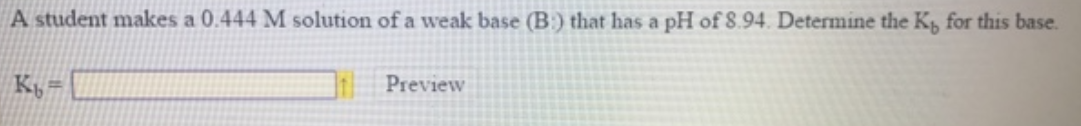Chemistry Practice Problems Weak Bases Practice Problems Solution: A student makes a 0.444 M solution of a weak base ...

# Solution: A student makes a 0.444 M solution of a weak base (B:) that has a pH of 8.94. Determine the Kb, for this base.

###### Problem

A student makes a 0.444 M solution of a weak base (B:) that has a pH of 8.94. Determine the Kb, for this base.View Complete Written Solution

Weak Bases

Weak Bases

#### Q. Calculate the pH and concentrations of CH3NH2 and CH3NH3+ in a 0.0343 M methylamine (CH3NH2) solution. The Kb of CH3NH2 = 4.47 x 10-4. pH= [CH3NH2] = ...

Solved • Tue Aug 14 2018 12:25:30 GMT-0400 (EDT)

Weak Bases

#### Q. If the Kb of a weak base is 5.4 x 10-6, what is the pH of a 0.50 M solution of this base?

Solved • Tue Aug 07 2018 17:52:17 GMT-0400 (EDT)

Weak Bases

#### Q. Part ACalculate the molar concentration of OH- ions in a 7.6 x 10-2 M solution of ethylamine (C2H5NH2) (Kb = 6.4 x 10-4). Express your answer using tw...

Solved • Tue Jul 24 2018 11:53:39 GMT-0400 (EDT)

Weak Bases

#### Q. If the Kb of a weak base is 35 x 10 -6, what is the pH of a 0.26 M solution of this base?

Solved • Mon Jul 09 2018 14:56:38 GMT-0400 (EDT)